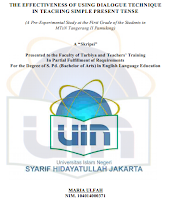# Skripsi gratis: using dialogue technique in teaching simple presenttenseDownload Skripsi Pendidikan Bahasa Inggris: using dialogue technique in teaching simple present tense

Judul Skripsi: The effectiveness of using dialogue technique in teaching simple present tense: a pre-experimental study at the first grade of the students in MTsN Tangerang II Pamulang
Research Design/Method: pre-experimental method
Research Instrument: test

Abstract
The purpose of this study is to describe the objective condition of the effectiveness of using dialogue technique in teaching simple present tense at the first grade of Islamic junior high school. The aim of the research is to gain the information about the effectiveness of dialogue technique in reinforcing the understanding of the simple present tense. Especially it is conducted to get the effective technique in teaching simple present tense at the first grade of the students in MTsN Tangerang II Pamulang in Bina prestasi class 2. the sample of the research was 24 students of the first grade students of MTsN Tangerang II Pamulang. This research using preexperimental method of the quantitative form by collecting data from observation and test. The data collected in this research were analyzed by using t-test. According to the result of statistical calculation, it was obtain the value of to (t observation) that 5.46 and the value of “tt” (t table) from the df (24) on the degree of significance of 5% is 2.07. it means that the value of to is higher than the value of tt. Based on the result the null hypothesis (Ho) which says there is no significance influence of using dialogue technique in simple present tense is rejected. It means that the alternative hypothesis (ha) which says there is significance influence of using dialogue technique in teaching simple present tense is accepted. Based on the finding of this study, it can be concluded that dialogue technique is effective and quite success because it can lead the students to be able to learn simple present tense well.